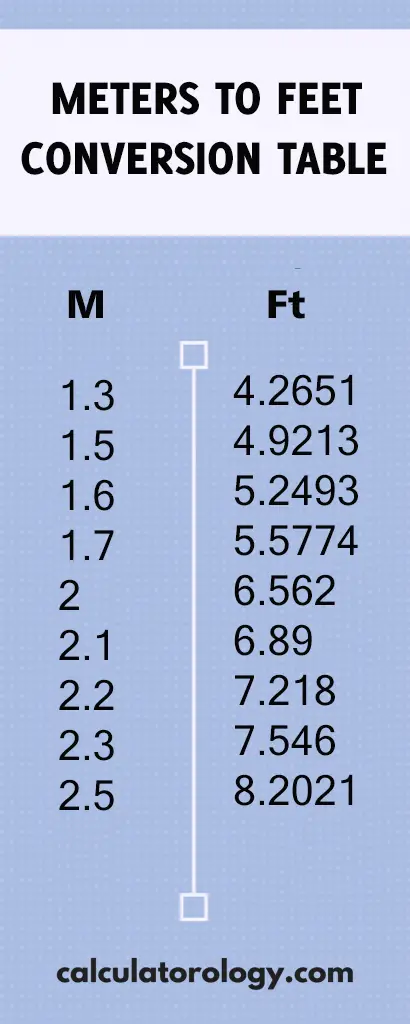код 2.5 meters to feet - Calculatorology

## 8.2020997375 feet

### 2.5 meters to feet

This conversion calculator is used to convert the distance in meters (m) to feet (ft.). It uses a straightforward formula to execute the conversions and give accurate results based on the input. If you want to find out the number of feet that are in 2.5 meters, it is important to determine how many feet make up one meter.

#### How many feet in 1 meter?

The conversion factor of meters to feet is 3.2808399. Multiply the value in meters by 3.2808399 to determine the number of feet in meters.

1 meter = 3.2808399 feet

By definition, 30.48 centimeters is equivalent to 1 foot and there are 100 centimeters in one meter. This translates to;

100/30.48 = 3.2808399 feet in a meter.## 2.5 Meter to Feet Conversion

 Meter Feet

#### How to convert 2.5 meters to feet

1 m = (1/0.3048) ft. = 3.28084 ft.

Therefore, 1 meter is equivalent to 3.28084 feet

D (ft.) = D (m) / 0.3048

The distance in feet is calculated by dividing the distance in meters by 0.3048.

For example;

Convert 2.5 meters to feet

Solution:

D (ft.) = 2.5 (m) / 0.3048

= 8.2020997375 feet

This means that there are 8.2020997375 feet in 2.5 meters.

The conversion calculator can be used to perform more calculations using different values. The ‘Reset’ button is used to erase all information of the previous calculations to create a new platform for other calculations. It can only perform a single conversion at a time.

2.5 meters to feet can also be determined using the Meters to Feet conversion table. It gives the conversion results of meters to feet based on a range of 0.01m to 100m.

##### Definitions

A meter is a length unit in the metric system defined as the distance travelled by light in 1/299792458 seconds. 1 meter is equivalent to 1.09 yards, 3.28 feet and 39.37 inches. It is also abbreviated as “m”

A foot is a length unit in the US customary and imperial systems of measurement abbreviated as “ft.” 1 foot is equivalent to 0.3048 meters.